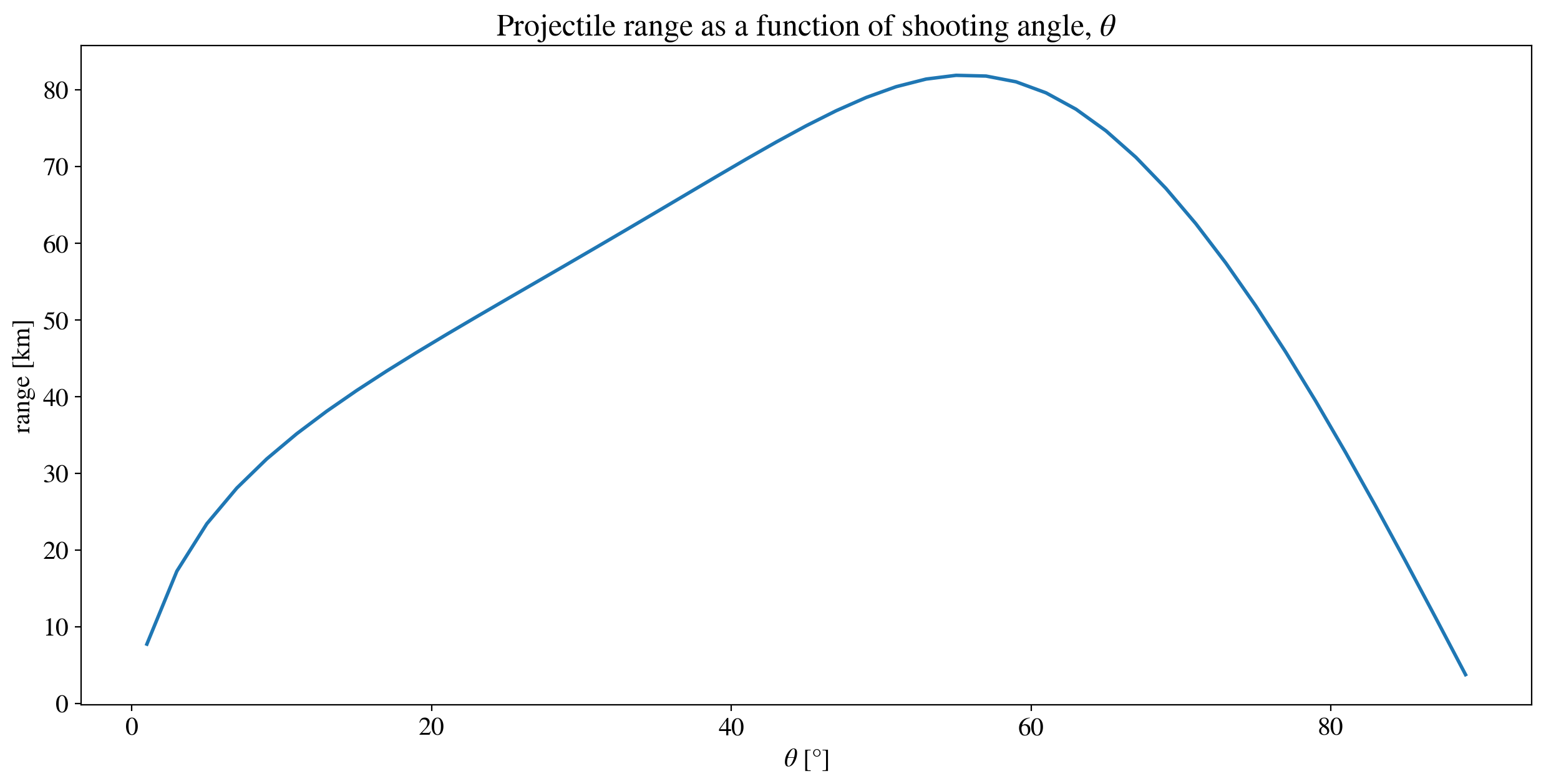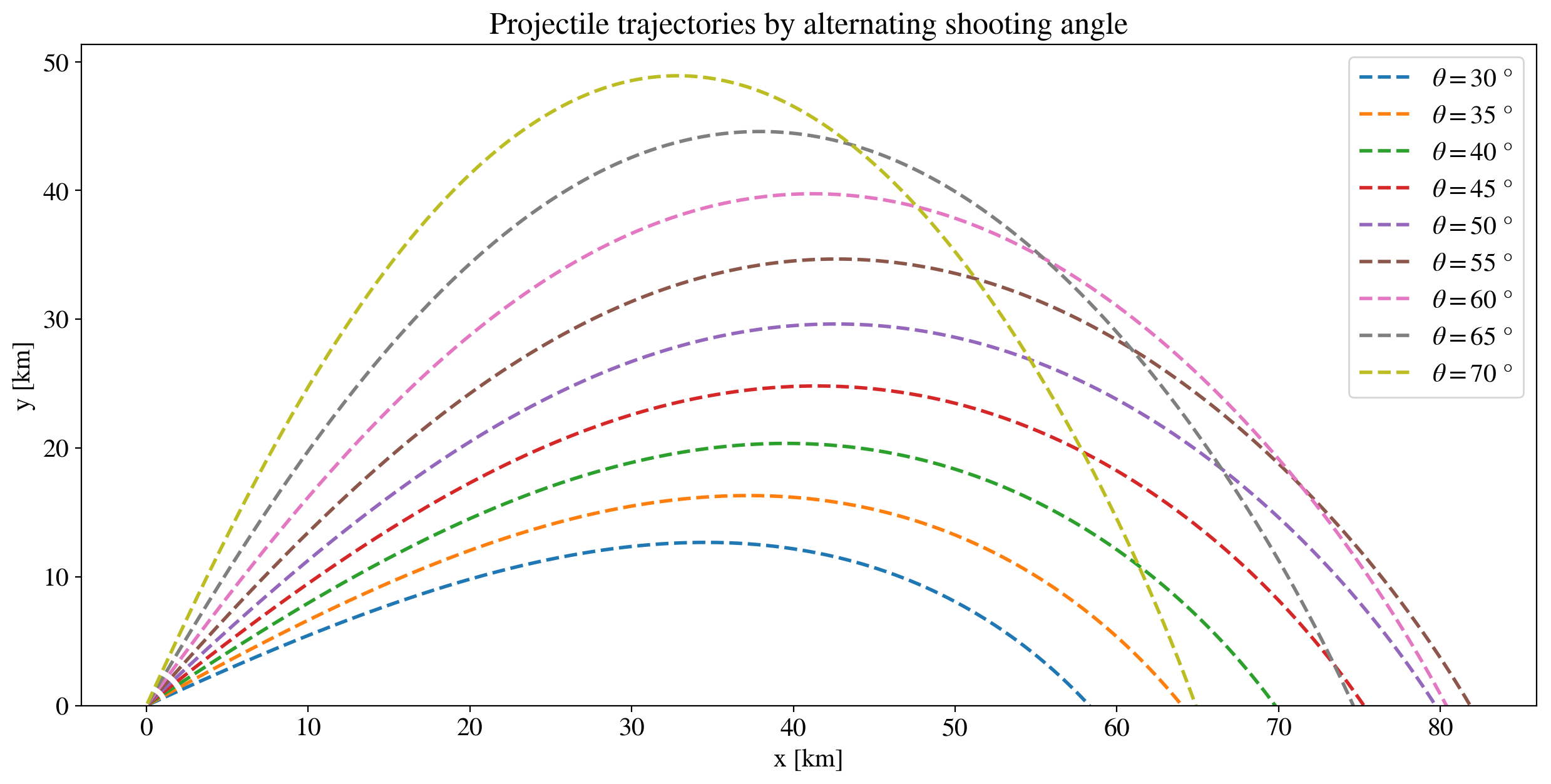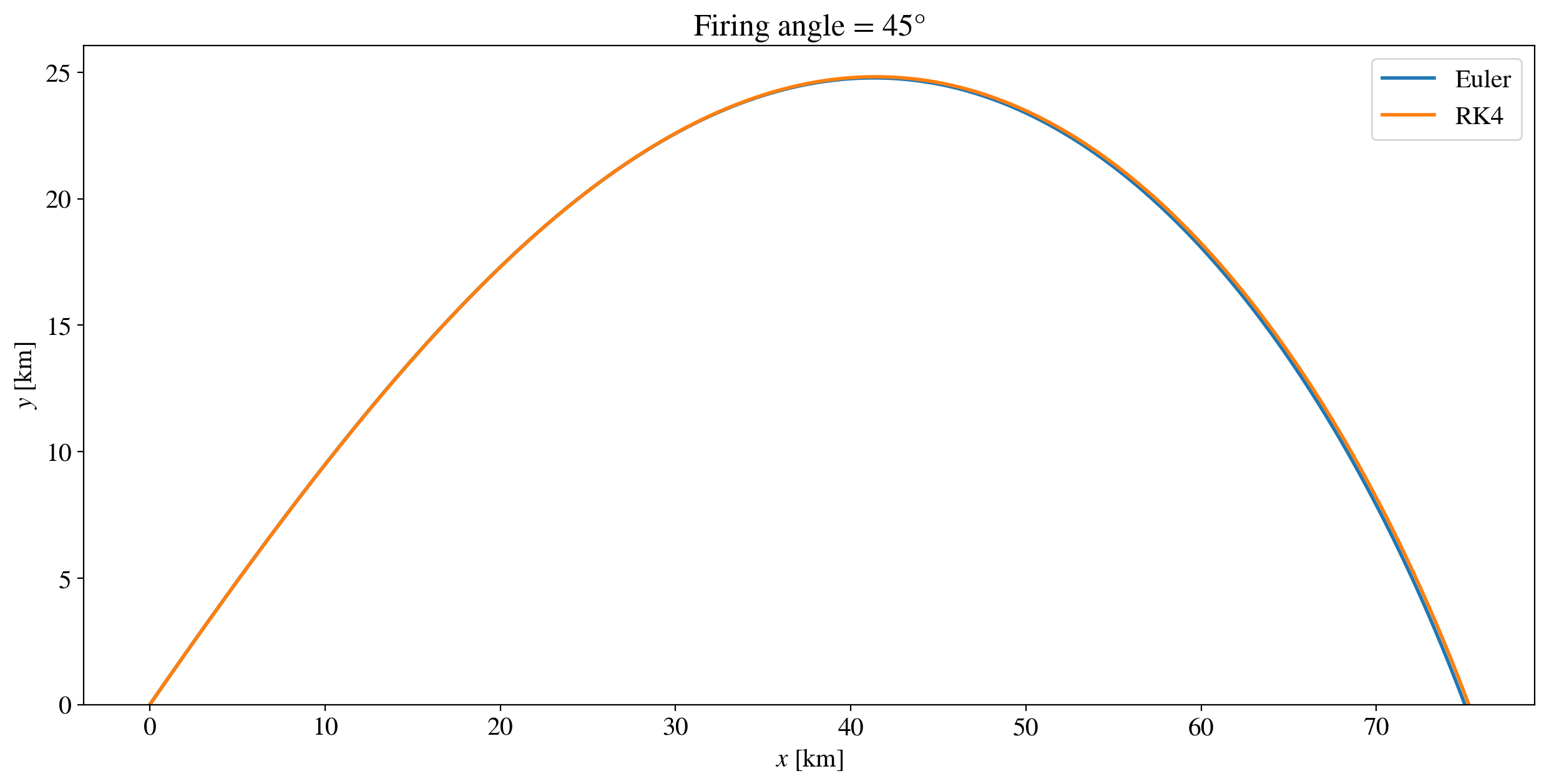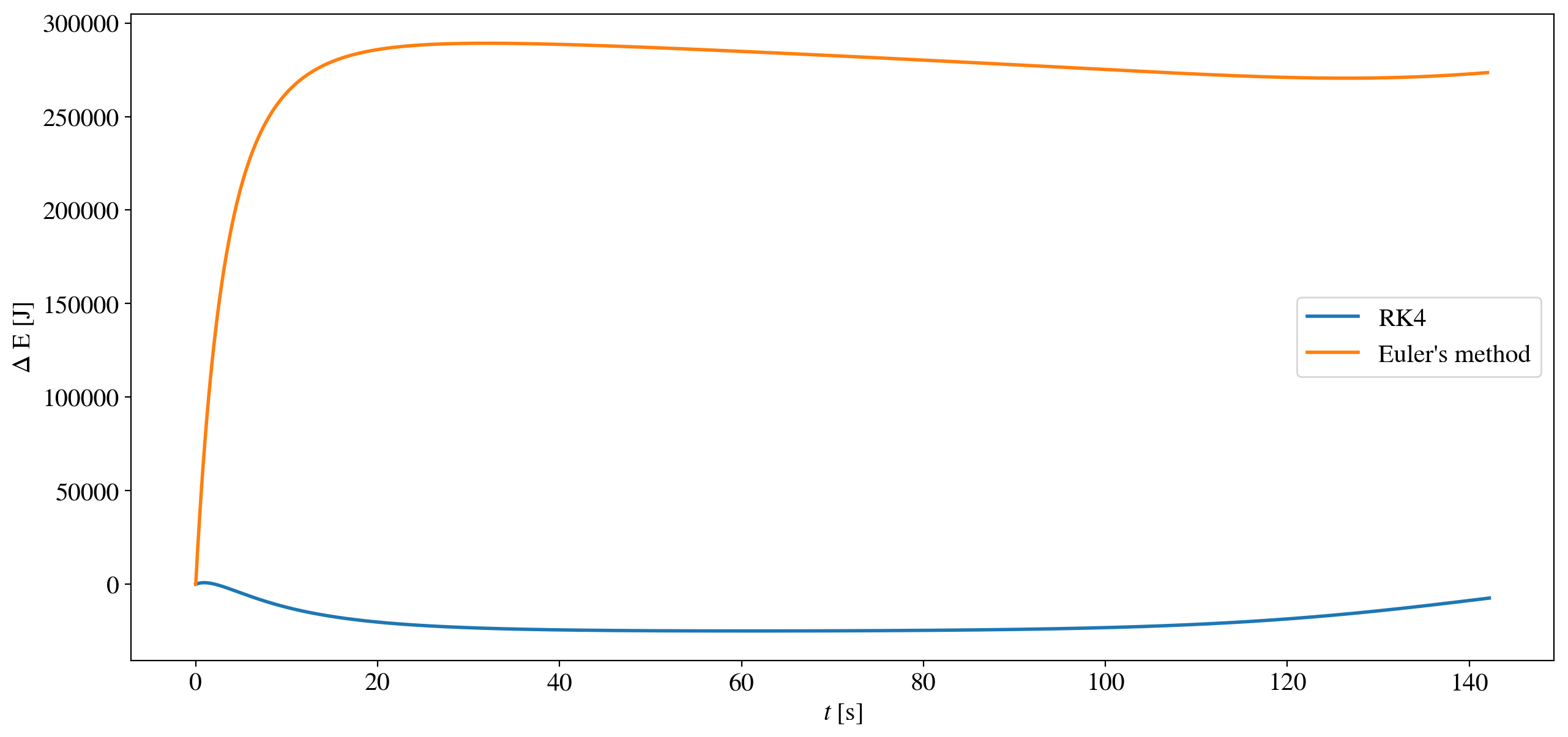# Shooting a projectile into the air¶

## Examples - Mechanics¶

Last edited: February 20th 2019

This notebook is based on a project assignment  given in the course TFY4245 Classical Mechanics at NTNU, fall 2018.

## Introduction¶

The trajectory of a projectile in a uniform gravity field is a classic and dear physics problem. However, in most introductory physics courses, one works under the assumption that the frictional forces can be neglected. This is of course not always the case, especially not at high speeds. If frictional forces is included, the path of the projectile can no longer be described analytically, and the trajectory must be calculated numerically. To do this, one must solve a coupled set of differential equations, which provides us with an excellent opportunity to implement an ODE-solver on vector form. This turns out to be an elegant and easy implementation, that can be used for solving both differential equations of higher order and coupled differential equations.

## Theory¶

### Equations of motion¶

The governing equations for a projectile is derived from Newtons second law, $\vec{F} = m\vec{a}$, where $\vec{F}$ is the force acting on the projectile, $m$ is the mass, and $\vec{a}$ is the acceleration of the ball. In this example there are two forces acting on the ball. The gravitational force

$$\vec{F_G} = m\vec{g},$$

where $\vec{g}$ denotes the the standard gravity. The other force is the drag force

$$\vec{F_D} = -\frac{1}{2}\rho ACv_r^2\hat{v_r}.$$

$A$ is the cross-sectional area, $C$ is the drag coefficient, and $v_r$ is the velocity of the projectile relative to the fluid it is traveling through, $\rho$ is the air density and $\hat{v_r}$ is the direction of the projectile with length unity. A more practical way of writing this equation is

$$\vec{F_D} = - \frac{B}{\rho_0}\rho v_r\left(\vec{v}-\vec{V}\right),$$

where $\rho_0$ is the air density at sea level altitude, $B$ is some constant, $\vec{v}$ is the velocity of the projectile, and $\vec{V}$ is the velocity of the wind.

In this example we will use the shells from the German cannon "the Paris gun" as the projectiles, and the numerical constants mentioned above will be based on historical data of the cannon. The Paris gun operated during World War I and bombarded Paris from far away, the distances often exceeded 100 kilometers, with the velocities of the shells being correspondingly high.

The complete equation for the drag force includes an extra term, which is not mentioned above, namely the term originating from Stokes' law. For a spherical projectile the term takes the form

$$\vec{F_D'} = -6 \pi \eta \vec{v},$$

where $\vec{F_D'}$ denotes the extra term, and $\eta$ denotes the air's viscosity. At high velocities this term is dwarfed by $\vec{F_D}$ as $\vec{F_D}$ is proportional to $v^2$, thus making the drag force $\vec{F_D}$ an excellent approximation for the total drag force.

The last part of the theory needed before we can find the equations of motion is an expression for the air density. As the shells of the Paris gun will reach the stratosphere, it is not reasonable to treat $\rho$ as a constant. In this notebook we will use an adiabatic air density model. The air density is then given by

$$\rho = \rho_0 \left(1 - \frac{ay}{T_0}\right)^\alpha,$$

where $\rho$ and $T_0$ denotes the air density and temperature at sea level, $y$ denotes the altitude, $a$ and $\alpha$ are constants $a= 6.5 \cdot 10^{-3}$ K/m, $\alpha$ = 2.5. At high altitudes the expression in the parentheses will become negative, thus making the air density an imaginary number. To avoid this, the air density is set equal to zero when reaching these altitudes. This critical height is around 44 kilometers, well into the stratosphere.

In a two-dimensional coordinate system, where $x$ and $y$ are the displacement from the origin in horizontal and vertical direction respectively, the equations of motion become \begin{align} m\ddot{x} &= -\frac{B}{\rho_0}\rho\left(\dot{x}-V_x\right)\left|\vec{v}-\vec{V}\right|,\\ m\ddot{y} &= -\frac{B}{\rho_0}\rho\left(\dot{y}-V_y\right)\left|\vec{v}-\vec{V}\right|-mg, \end{align}

where $V_x$ and $V_y$ denotes the velocity of the wind in the horizontal and vertical direction. Simplifying even further, and introducing $u$ and $v$ as the velocities in the horizontal and vertical direction yields four coupled differential equations

\begin{equation} \begin{aligned} \dot{x} &= u, \\ \dot{u} &= -\frac{B}{m}\Big[ 1 - \frac{ay}{T_0} \Big]^\alpha \left(u-V_x\right)\Big[ (u-V_x)^2+(v-V_y)^2 \Big]^{1/2},\\ \dot{y} &= w, \\ \dot{w} &= -\frac{B}{m}\Big[ 1 - \frac{ay}{T_0} \Big]^\alpha \left(v-V_y\right)\Big[ (u-V_x)^2+(v-V_y)^2 \Big]^{1/2}-g.\\ \end{aligned} \label{ODEs} \end{equation}

### Discretization: Runge-Kutta 4th order¶

First we define a set of $N$ discrete time values $$t_n=t_0+n\cdot h~~~~~\mathrm{with}~~~~n=0,1,2,3,...,N.$$

$h$ is the time step length and is calculated by the formula $h = \frac{t_N-t_0}{N}$, where $t_0$ and $t_N$ denotes the initial and final time values of the projectile's flight. These $N$ time values correspond to $N$ number of $x$, $y$, $u$, and $w$ values, each denoted $x_n$, $y_n$, $u_n$ and $w_n$. A more practical way of denoting this collection of values is on vector form $$\vec{w_n} = \begin{bmatrix}x_n \\ y_n \\ u_n \\ v_n\end{bmatrix}.$$

This notation makes it possible to implement the Runge-Kutta method in an elegant way. It is always possible to reduce an ordinary differential equation of order $q$ to a problem of solving a set of two $q-1$ order coupled differential equations. We have a coupled set of two second order differential equations, thus we need to solve a set of four first order coupled differential equations. By introducing a function $f$ that transforms $\vec{w_n}$ in the way described by equation \eqref{ODEs}, one can implement the Runge-Kutta method directly. In our case, $f$ is given by

$$f(\vec{w_n} ) = f \begin{bmatrix}x_n \\ y_n \\ u_n \\ v_n\end{bmatrix} = \begin{bmatrix}u_n \\ v_n \\ -\frac{B}{m}\Big[ 1 - \frac{ay_n}{T_0} \Big]^\alpha \left(u_n-V_x\right)\Big[ (u_n-V_x)^2+(v_n-V_y)^2 \Big]^{1/2} \\ -\frac{B}{m}\Big[ 1 - \frac{ay_n}{T_0} \Big]^\alpha \left(v_n-V_y\right)\Big[ (u_n-V_x)^2+(v_n-V_y)^2 \Big]^{1/2}-mg\end{bmatrix},$$

with no time dependence, such that $$\dot{\vec{w_n}}=f(\vec{w_n})$$

describes the complete system of differential equations. For further reading, check out the notebook on the Runge-Kutta method. The following code implements this, and can easily be expanded to solve either an even higher order ODE or an ODE describing a three-dimensional problem.

## Implementation¶

In :
# Importing necessary packages
import numpy as np
import matplotlib.pyplot as plt
from matplotlib import rc
%matplotlib inline

newparams = {'figure.figsize': (15, 7), 'axes.grid': False,
'lines.markersize': 10, 'lines.linewidth': 2,
'font.size': 15, 'mathtext.fontset': 'stix',
'font.family': 'STIXGeneral', 'figure.dpi': 200}
plt.rcParams.update(newparams)

# Constants

g = 9.81             # Force of gravity per kilo on earth's surface
alpha = 2.5          # Parameter in the adiabatic air density model
a = 6.5 * 10 ** (-3) # Parameter in the adiabatic air density model
T_0 = 288            # Temperature at sea level
m = 50               # Mass of the projectile
B = 2 * 10 ** (-3)   # Constant based on the Paris gun
v_0 = 1640           # Initial velocity

# Wind strength. This is a constant that never varies
V = np.zeros(2) # Starting of with no wind

def f(w):
""" A function describing the right hand side of the equations
of motion/the set of ODEs.
Parameter:
w       vector containg the needed coordinates and their velocities
"""
# V : the vector describing the wind strength and direction
temp_vector = np.zeros(4)
temp_vector = w
temp_vector = w
# Saving the next parameters in order to optimize run time
k = (1 - a * w / T_0)   # Air density factor
s = np.sqrt((w-V) ** 2 + (w-V) ** 2 )
if k>0:
temp_vector = - B / m * k ** (alpha) * (w-V) * s
temp_vector = - B / m * k ** (alpha) * (w-V) * s - g
else:
temp_vector = 0
temp_vector = - g
return temp_vector

def RK4_step(f, w, h):
"""Performing a single step of the Runge-Kutta fourth order method.
Parameters:
f      RHS of ODEs to be integrated
w      numerical approximation of w at time t
h      unit/integration step length
Returns:
numerical approximation of w at time t+h
"""
s1 = f(w)
s2 = f(w + (h / 2) * s1)
s3 = f(w + (h / 2) * s2)
s4 = f(w + h * s3)
return w + (h / 6) * (s1 + (2 * s2) + (2 * s3) + s4)


Now we can finally start to explore the trajectories of the Paris gun. The functions below will be helpful going forward.

In :
def shoot(theta, v0):
""" Initializes the vector w (x and y position and velocity of the
projectile) given a initial shooting angle, theta, and
absolute velocity, v0.
"""
w = np.zeros(4)
return w

def projectile_motion(h, theta):
""" Calculates the motion of the projectile using the functions
defined above. While the projectile is in the air (w >=0) the
position and velocity is updated using a single step of RK4.
Parameters:
h        unit/integration step length
theta    initial shooting angle
Returns:
X_list   array of the projectile's x-position
Y_list   array of the porjectile's y-position
"""
w = shoot(theta, v_0)
X_list = np.zeros(0)
Y_list = np.zeros(0)
while  w >= 0:
w = RK4_step(f, w, h)
X_list = np.append(X_list, w)
Y_list = np.append(Y_list, w)
return X_list, Y_list


### Finding the optimal launch angle¶

With no drag force, it is trivial to show that the angle maximizing the range of a projectile is $\theta = 45^\circ$, where $\theta$ is the angle between the horizontal axis and the direction of the initial velocity. It is interesting to se how the air drag affects this maximum angle, both with and without wind. The following code finds the optimal angle to one decimal precision, and shows how the range depends on the firing angle. It is also instructive to see some of the trajectories of the projectile, so some of the trajectories are included.

In :
def find_optimal_angle(h):
""" Given an integration time step, this function calculates the optimal initial
shooting angle for the projectile to obtain maximum range, in x-direction. The
set of angles tested, with their corresponding range, along with the optimal
angle are returned.
"""

record = 0        # Placeholder variable that holds the maximum range
optimal_angle = 0 # Placeholder variable that holds the angle yielding the maximum range
# Lists containing the initial angle and its corresponding range
theta_list = np.zeros(0)
range_list = np.zeros(0)
for theta in range (1,90,2):
x_list, y_list = projectile_motion(h, theta)
# Using linear interpolation do determine the landing point more precisely
m = (y_list[-1] - y_list[-2]) / (x_list[-1] - x_list[-2])   # The landing point
x_range = - y_list[-1] / m + x_list[-1]
theta_list = np.append(theta_list, theta)
range_list = np.append(range_list, x_range)
# Update records
if x_range >= record:
record = x_range
optimal_angle = theta

# Rerunning the same code on a smaller interval in order to approximate the optimal angle
# more precicely
theta_list_smaller = np.linspace(optimal_angle - 2, optimal_angle + 2, 41)
for theta_small in theta_list_smaller:
x_list, y_list = projectile_motion(h, theta)
# Again, using linear interpolation do determine the landing point more precisely
m = (y_list[-1] - y_list[-2]) / (x_list[-1] - x_list[-2])
x_range = - y_list[-1] / m + x_list[-1]
if x_range >= record:
record = x_range
optimal_angle = theta_small

return theta_list, range_list, optimal_angle

theta, x , best = find_optimal_angle(0.1)

print("The optimal angle is: ", best, " degrees")

The optimal angle is:  55  degrees

In :
plt.plot(theta, x/1000)
plt.title(r"Projectile range as a function of shooting angle, $\theta$")
plt.xlabel(r"$\theta$ [$\degree$]")
plt.ylabel(r"range [km]")

def trajectories(h):
plt.figure()
plt.title("Projectile trajectories by alternating shooting angle")
plt.xlabel(r"x [km]")
plt.ylabel(r"y [km]")
theta_list = np.arange(30.0,75,5)
for angle in theta_list:
x_list, y_list = projectile_motion(h, angle)
plt.plot(x_list/1000, y_list/1000, '--', label=r'$\theta =$%.i $\degree$'%(angle))
plt.legend(loc='best')
plt.gca().set_ylim(bottom=0)
plt.show()
trajectories(0.1)In contrast to projectile motion with no friction, maximum range is not obtained with firing angle $\theta = 45^\circ$. Because the drag force is lower at higher altitudes, the range increases when the projectile spends the majority of it's flight at higher altitudes. This explains why the maximum range is obtained at $\theta = 55^\circ$.

### Numerical validity¶

In order to determine the validity of the numerical implementation, we can use conservation of energy. During the projectile's trajectory the drag force $\vec{F_D}$ will do work on the projectile. The cumulative work done on the projectile is given by

$$W = \int \vec{F_D} \cdot \vec{dr}.$$

In our discrete model, we can easily calculate this integral by summing over all the infinitesimal contributions, and using the fact that $\vec{F_D}$ always is antiparallel with $\vec{dr}$ such that the cumulative work at time $t_M$, $M<N$, is equal to

$$W = \sum_{n=1}^{M} |\vec{F_{D_{n-1}}}| \cdot |\vec{r_n} - \vec{r_{n-1}}|,$$

where $\vec{F_{D_{n-1}}}$ denotes the drag force at position $\vec{r_{n-1}}$. However, this can again be written to the form

$$W = h \sum_{n=1}^{M} |\vec{F_{D_{n-1}}}| \cdot |\vec{v_{n-1}}|,$$

which is the most convenient for our purposes. It must be noted that the choice of index that is used for the force and speed respectively is non-trivial, as they both will vary during the interval $dr$. Here both the speed of the projectile and the force at point $\vec{r_{n-1}}$ is used. This is mainly a pragmatic choice, as this combination yields the lowest, and thus the most realistic energy loss. Thus we can finally express the energy of the projectile (which of course is equal to it's kinetic and potential energy) and the work done on the air at time $t_M$

$$E(t_M) = h \sum_{n=1}^{M} |\vec{F_{D_{n-1}}}| \cdot |\vec{v_{n-1}}| + \frac{1}{2} m v_m^2 + mgy_m,$$

where $v_m$ is the absolute velcoity of the projectile, and $y_m$ is the height of the projectile over the ground (zero potential reference point).

Now all that remains is to check if this expression for the energy indeed remains constant while it is in the air.

In this notebook, the Runge-Kutta method is implemented without much difficulties. However, it is interesting to see whether or not it was necessary to use a 4th order differential equation solver, instead of using the most primitive ODE solver, Euler's method. The following code will use both Runge-Kutta and Euler's method, and compare the energy loss during the motion for both of them.

In :
def euler_step(f ,w ,h):
"""Simple implementation of Euler's method on vector form
"""
return w + h * f(w)

def energy_conservation(h, theta, func):
""" This function performs the same steps as "projectile_motion()" and "f()"
but extracts the value for the drag, F_D, at every step. This enables the
calculation of work and storage of energy at each time step.
Parameters:
h           integration time step
theta       initial shooting angle
func        a function defining the integration step (euler or RK4)
Returns:
x_list      array of the trajectory x-coordinates
y_list      array of the trajectory y-coordinates
time_steps  array of the elapsed time at each step
energy      array of the energy at each step
work        the total work by the drag force
"""
w = shoot(theta, v_0)

x_list = np.zeros(1)
y_list = np.zeros(1)
work = 0

# Initial total energy
v = np.sqrt(w ** 2 + w ** 2)
energy = np.array([0.5 * m * v ** 2])

while w >= 0:
w = func(f, w, h)

# Updating lists
x_list = np.append(x_list, w)
y_list = np.append(y_list, w)

# Temporary parameters for checking if high altitude
k = (1 - a * y_list[-2] / T_0)
s = np.sqrt((w - V) ** 2 + (w - V) ** 2)
if k > 0:
F_D = B * k ** (alpha) * s ** 2
else:
F_D = 0

# Add the amount of work done by drag this time interval
work +=  v * F_D * h
# Updating the speed in order to calculate the total energy
v = np.sqrt(w ** 2 + w ** 2)
# Use np.sum on the work_list to get the cumulative work
energy = np.append(energy, 0.5 * m * v ** 2 + m * g * w + work)

n = len(x_list)
time_steps = np.linspace(0, n * h, n)

return x_list, y_list, time_steps, energy, work

# Setting initial values
theta = 45
h = 0.1
# Getting the results
x_list_euler, y_list_euler, time_steps_euler, energy_euler, work = energy_conservation(h, theta, euler_step)
x_list_RK4, y_list_RK4, time_steps_RK4, energy_RK4, work = energy_conservation(h, theta, RK4_step)

print("At firing angle %.1f degrees and with time step h = %.2f seconds:" %(theta, h))
print("Energy lost with RK4: %.3E = %.2f percent of total energy" %(energy_RK4 - energy_RK4[-1],
(energy_RK4 - energy_RK4[-1]) / energy_RK4 * 100))
print("Energy lost with Euler's method: %.3E = %.2f percent of total energy" %(energy_euler - energy_euler[-1],
(energy_euler - energy_euler[-1]) / energy_euler * 100))

# Plotting the separate trajectories
plt.plot(x_list_euler/1000, y_list_euler/1000, label ="Euler")
plt.plot(x_list_RK4/1000, y_list_RK4/1000, label ="RK4")
plt.title("Firing angle = " + str(theta) + "$\degree$")
plt.xlabel(r"$x$ [km]")
plt.ylabel(r"$y$ [km]")
plt.gca().set_ylim(bottom=0)
plt.legend()
plt.show()

At firing angle 45.0 degrees and with time step h = 0.10 seconds:
Energy lost with RK4: -7.395E+03 = -0.01 percent of total energy
Energy lost with Euler's method: 2.735E+05 = 0.41 percent of total energyFrom the figure above, it is clear that Euler's method work surprisingly well, even though it us just ODE-solver of first order. It produces nearly the same trajectory as Runge Kutta 4th method. The most likely explanation of the small difference between Runge Kutta 4th order and Euler's method is that the coupled set of ODEs we are solving are quite linear, in other words, the derivatives vary quite slowly. Thus Euler's method produce satisfactory results, even though it is only of first order.

It might seem like loosing around $10^3$ joules is a lot, but one needs to remember the enormous dimensions of the Paris gun, and that this is a small fraction of the original energy of the projectile.

## The physics behind the numerics¶

Even though the amount of disappeared energy is relatively small, it is still interesting to study the nature of the loss. In our case it turns out that it has an explanation that has roots in both the physics and the numerics of the problem.

In :
# When does the energy disappear during the flight?
starting_energy_RK4 = np.repeat(energy_RK4, len(energy_RK4))
starting_energy_euler = np.repeat(energy_euler, len(energy_euler))

plt.plot(time_steps_RK4, starting_energy_RK4 - energy_RK4, label = "RK4")
plt.plot(time_steps_euler, starting_energy_euler - energy_euler, label = "Euler's method")
plt.legend()
plt.xlabel(r"$t$ [s]")
plt.ylabel(r"$\Delta$ E [J]")

plt.show()

# Exploring the numerical validity as a function of the firing angle
energy_loss_euler = np.zeros(0)
energy_loss_RK4 = np.zeros(0)
angles = np.zeros(0)
for i in range(90):
energy_euler = energy_conservation(0.1, i, euler_step) # only returns the fourth return value
energy_loss_euler = np.append(energy_loss_euler, energy_euler - energy_euler[-1])
energy_RK4 = energy_conservation(0.1, i, RK4_step)
energy_loss_RK4 = np.append(energy_loss_RK4, energy_RK4 - energy_RK4[-1])
angles = np.append(angles, i)

plt.plot(angles, energy_loss_RK4, label ="RK4")
plt.plot(angles, energy_loss_euler, label ="Euler's method")
plt.legend()
plt.xlabel(r"$\theta$ [$\degree$]")
plt.ylabel(r"$\Delta$ E [J]")
plt.show()